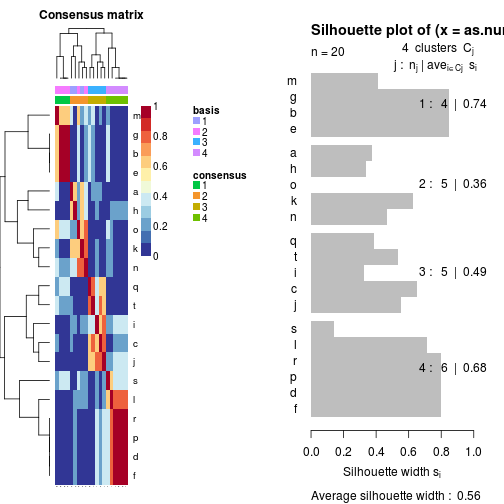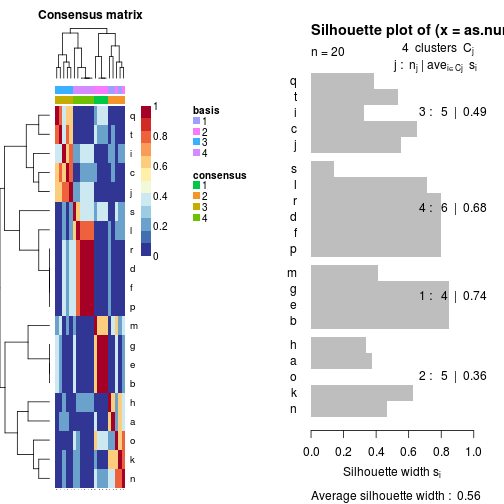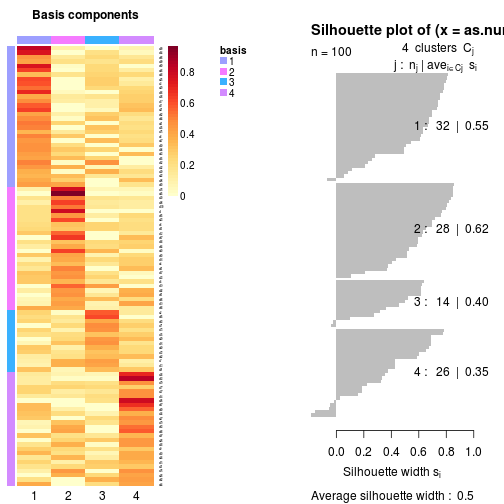# Silhouette of NMF Clustering

## Description

Silhouette of NMF Clustering

## Usage

```S3 (NMF)
`silhouette`(x, what = NULL, order = NULL, ...)```

## Arguments

x
an NMF object, as returned by `nmf`.
what
defines the type of clustering the computed silhouettes are meant to assess: `'samples'` for the clustering of samples (i.e. the columns of the target matrix), `'features'` for the clustering of features (i.e. the rows of the target matrix), and `'chc'` for the consensus clustering of samples as defined by hierarchical clustering dendrogram, `'consensus'` for the consensus clustering of samples, with clustered ordered as in the default hierarchical clustering used by `consensusmap` when plotting the heatmap of the consensus matrix (for multi-run NMF fits). That is ```dist = 1 - consensus(x)```, average linkage and reordering based on row means.
order
integer indexing vector that can be used to force the silhouette order.
...
extra arguments not used.

## Examples

``````
x <- rmatrix(100, 20, dimnames = list(paste0('a', 1:100), letters[1:20]))
res <- nmf(x, 4, nrun = 5)

# sample clustering from best fit
plot(silhouette(res))
````````````
# from consensus
plot(silhouette(res, what = 'consensus'))
````````````
# feature clustering
plot(silhouette(res, what = 'features'))
````````````
# average silhouette are computed in summary measures
summary(res)
``````
``````##   Length    Class     Mode
##        1 NMFfitX1       S4
``````
``````
# consensus silhouettes are ordered as on default consensusmap heatmap
op <- par(mfrow = c(1,2))
consensusmap(res)
si <- silhouette(res, what = 'consensus')
plot(si)
````````````par(op)

# if the order is based on some custom numeric weights
op <- par(mfrow = c(1,2))
cm <- consensusmap(res, Rowv = runif(ncol(res)))
# NB: use reverse order because silhouettes are plotted top-down
si <- silhouette(res, what = 'consensus', order = rev(cm\$rowInd))
plot(si)
````````````par(op)

# do the reverse: order the heatmap as a set of silhouettes
si <- silhouette(res, what = 'features')
op <- par(mfrow = c(1,2))
basismap(res, Rowv = si)
plot(si)
````````````par(op)

``````

`predict`